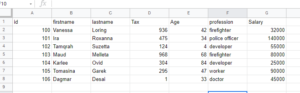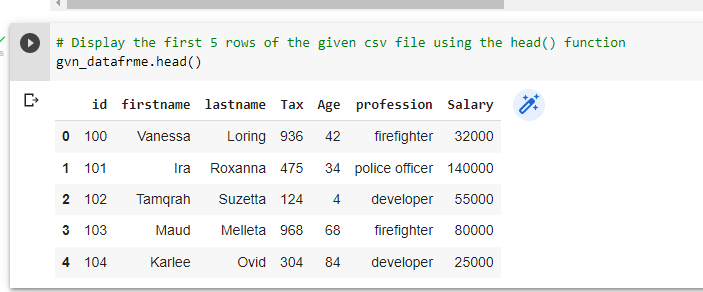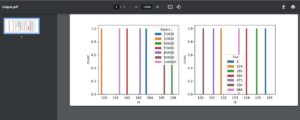# How to Save Multiple matplotlib Figures in Single PDF File in Python?

In this article, we’ll learn how to save several plots in a single pdf file. In many scenarios, we need our output to be in a specific format, which we can easily acquire in Python by utilizing the following method.

Let us take the demo.csv file as an example here to perform the task.## Saving Multiple matplotlib Figures in Single PDF File in Python

1)Importing libraries

# Import pandas module using the import keyword
import pandas as pd
# Import pyplot from matplotlib module using the import keyword
from matplotlib import pyplot as pplot
# Import seaborn module using the import keyword
import seaborn as sns


# Pass some random CSV file to the read_csv() function of the pandas module
# to read the given csv file
gvn_datafrme = pd.read_csv("demo.csv")

3)Displaying the first 5 rows of the CSV file

# Display the first 5 rows of the given csv file using the head() function
gvn_datafrme.head()

Output:4)Plotting Multiple plots of a Given CSV File in a Single PDF File

# Passing some random plot size(width, length) to the figure() function
pplot.figure(figsize=(10, 4))
# Plot the subplot by passing the arguments rows, columns and order of the plot to the
# subplot() function
pplot.subplot(1, 2, 1)
# Plot the graph of id, Salary columns in a given CSV file using the countplot() function
# of the seaborn module
sns.countplot('id', hue='Salary', data= gvn_datafrme)
pplot.subplot(1, 2, 2)
# Plot the graph of id, Tax columns in a given CSV file using the countplot() function
# of the seaborn module
# Here we are plotting multiple plots in a single PDF file.
sns.countplot('id', hue='Tax', data= gvn_datafrme)
# Save the plots in a single PDF file by passing some random name to the savefig() function
pplot.savefig('Output.pdf')

## Total Code

Approach:

• Import pandas module using the import keyword
• Import pyplot from matplotlib module using the import keyword
• Import seaborn module using the import keyword
• Pass some random CSV file to the read_csv() function of the pandas module to read the given csv file
• Display the first 5 rows of the given csv file using the head() function
• Passing some random plot size(width, length) to the figure() function
• Plot the graph of id, Salary columns in a given CSV file using the countplot() function of the seaborn module
• Plot the subplot by passing the arguments rows, columns and order of the plot to the subplot() function
• Plot the graph of id, Tax columns in a given CSV file using the countplot() function of the seaborn module
• Here we are plotting multiple plots in a single PDF file.
• Save the plots in a single PDF file by passing some random name to the savefig() function.
• The Exit of the Program.

Below is the implementation:

# Import pandas module using the import keyword
import pandas as pd
# Import pyplot from matplotlib module using the import keyword
from matplotlib import pyplot as pplot
# Import seaborn module using the import keyword
import seaborn as sns
# Pass some random CSV file to the read_csv() function of the pandas module
# to read the given csv file
# Display the first 5 rows of the given csv file using the head() function
# Passing some random plot size(width, length) to the figure() function
pplot.figure(figsize=(10, 4))
pplot.subplot(1, 2, 1)
# Plot the graph of id, Salary columns in a given CSV file using the countplot() function
# of the seaborn module
sns.countplot('id', hue='Salary', data= gvn_datafrme)
# Plot the subplot by passing the arguments rows, columns and order of the plot to the
# subplot() function
pplot.subplot(1, 2, 2)
# Plot the graph of id, Tax columns in a given CSV file using the countplot() function
# of the seaborn module
# Here we are plotting multiple plots in a single PDF file.
sns.countplot('id', hue='Tax', data= gvn_datafrme)
# Save the plots in a single PDF file by passing some random name to the savefig() function
pplot.savefig('Output.pdf')

Output: